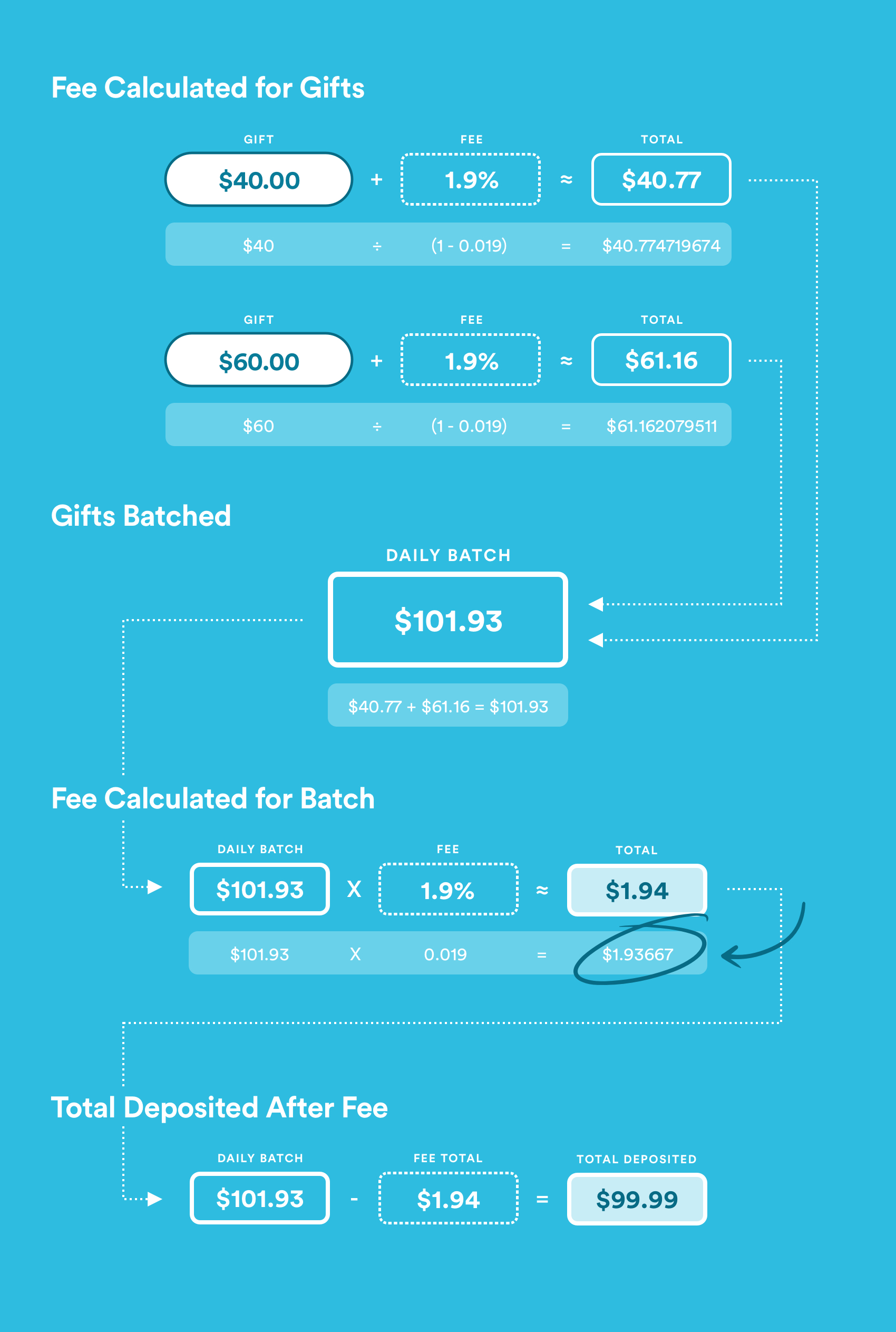# Why are my bank statements a penny off?

From time to time, you may notice that your bank statement is off by a penny in comparison to what you see in your Control Center. This is because all donations given via credit cards are charged a percentage transaction fee of 1.9%.

Here's an example, using two separate donations of \$40 and \$60, both given via credit card.Every gift donated through a GivingFlow goes through the calculation of x / (1 - 0.019), where x = gift amount. The result is the total amount the donor pays to account for the transaction fee.

For these two individual gifts, the calculations are as follows:

DONOR 1: \$40 / (1 - 0.019) = \$40.774719674
DONOR 2: \$60 / (1 - 0.019) = \$61.162079511

We cannot deposit partial pennies, however, so these amounts are rounded to the nearest whole penny. Thus, the following is how much each donor gives:

DONOR 1: \$40.774719674 ≈ \$40.77
DONOR 2: \$61.162079511 ≈ \$61.16

The idea is that when you multiply the total amount by 1.9% and then remove that small amount, you're left with the full amount the giver wanted to give.

DONOR 1: \$40.77 * 0.019 = \$0.77463 ≈ \$0.77
DONOR 2: \$61.16 * 0.019 = \$1.16204 ≈ \$1.16

DONOR 1: \$40.77 - \$0.77 = \$40
DONOR 2: \$61.16 - \$1.16 = \$60

Let's say, in this example, these two donations are the only two gifts given in a 24 period, meaning they are the only two transactions in the next batch deposit. Let's add the total of the two gifts with and without the transaction fees:

WITH FEES: \$40.77 + \$61.16 = \$101.93
WITHOUT FEES: \$40 + \$60 = \$100

Since the total of each donor's gift is \$100, intuitively it makes sense that the transaction fee given to the card processor is \$1.93. (See calculation below.)

TRANSACTION FEE (INTUITIVELY): \$101.93 - \$100 = \$1.93

This is the point where the discrepancy occurs. Each batch is deposited after the percentage fee is taken off the total batch amount. (Card processors do not send each transaction out to the credit card companies as they happen; they batch them daily.)

TRANSACTION FEE (ACTUALITY): \$101.93 * 0.019 = \$1.93667 ≈ \$1.94

Just like how each individual gift is rounded to the nearest penny, so is the total transaction fee of each batch deposit. In this case, it happens to be rounded up, leaving with a penny difference between what one would expect versus what actually occurs, due to credit card transaction fees being a percentage rather than a set flat rate.

PENNY DISCREPANCY: \$1.94 - \$1.93 = \$0.01

If only one credit card donation is made in a 24-hour period, there is no room for this discrepancy; however, as soon as there are multiple donations made, there is no way to answer the question, "Which donor do you choose to have a penny removed from their gift to account for the difference?"

In this example, there is a penny less than what adds up to the total amount of gifts deposited in that single batch; however, there may be other times where there will be a penny more. It all depends on whether the percentage transaction fee requires the card processor to round up or down by one penny.